• Размер: 460.5 Кб
• Количество слайдов: 13

## Описание презентации L 9 – Circular Motion • Be по слайдам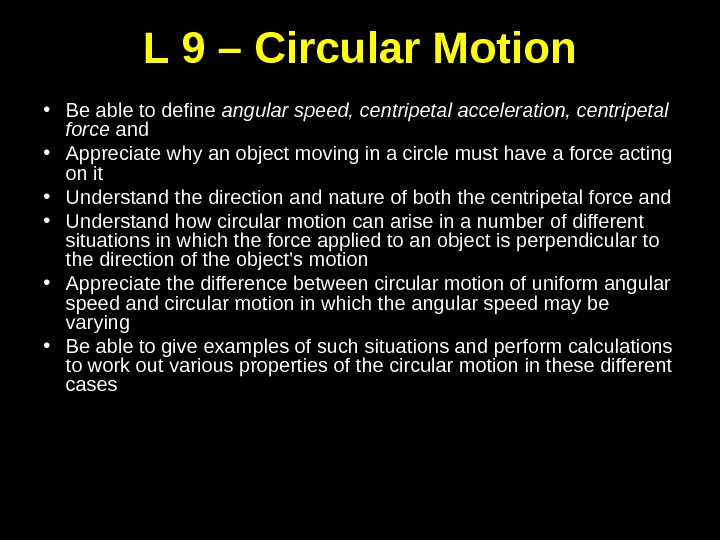L 9 – Circular Motion • Be able to define angular speed, centripetal acceleration, centripetal force and • Appreciate why an object moving in a circle must have a force acting on it • Understand the direction and nature of both the centripetal force and • Understand how circular motion can arise in a number of different situations in which the force applied to an object is perpendicular to the direction of the object’s motion • Appreciate the difference between circular motion of uniform angular speed and circular motion in which the angular speed may be varying • Be able to give examples of such situations and perform calculations to work out various properties of the circular motion in these different cases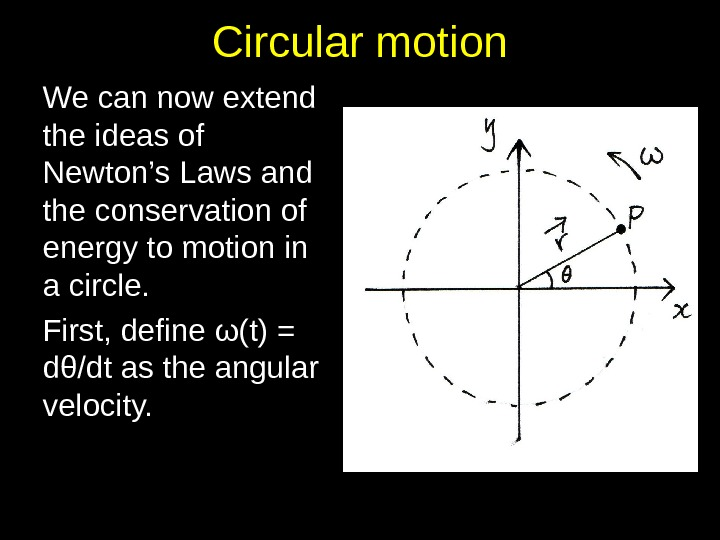Circular motion We can now extend the ideas of Newton’s Laws and the conservation of energy to motion in a circle. First, define ω(t) = dθ/dt as the angular velocity.Now imagine a particle, P, as the end po int of a position vector r moving with constant angular speed . If r(t) starts on the x-axis, then t sec onds later: r r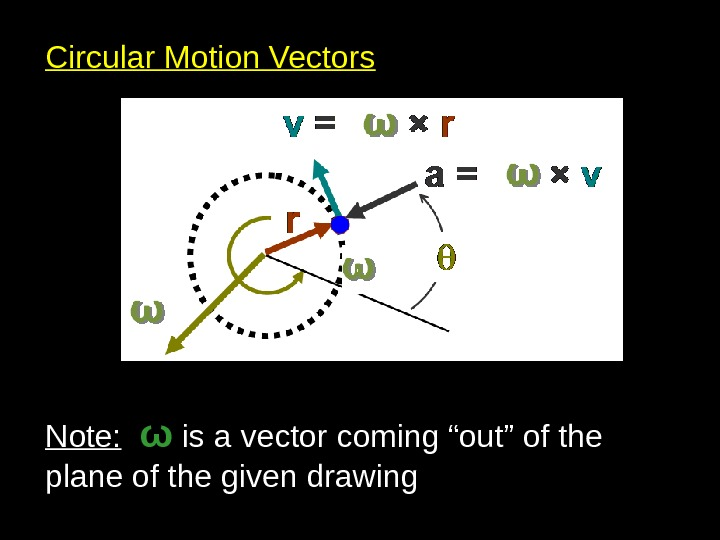Circular Motion Vectors Note: ω is a vector coming “out” of the plane of the given drawingSummary 2 2 2 For a particle moving with constant angu lar speed about a fixed point, O, whose posi tion vector r(t) = rcos t i + r sin t j: v(t) = r sin t i + r cos t j v=r a(t) = v r a r r r r rCentripetal force Clearly, if an object is accelerating there must be a force acting on it. The force which causes centripetal acceleration is called the centripetal force.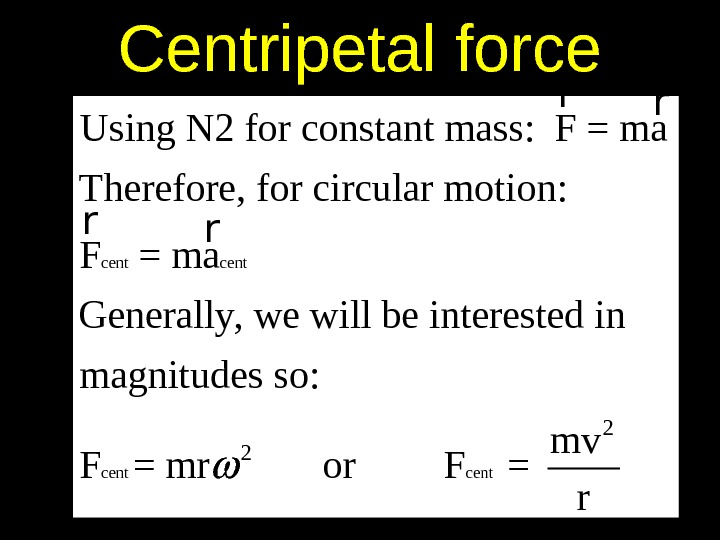Centripetal force cent 2 2 Using N 2 for constant mass: F = ma Therefore, for circular motion: F = ma Generally, we will be interested in magnitudes so: mv F = mr or F = rr r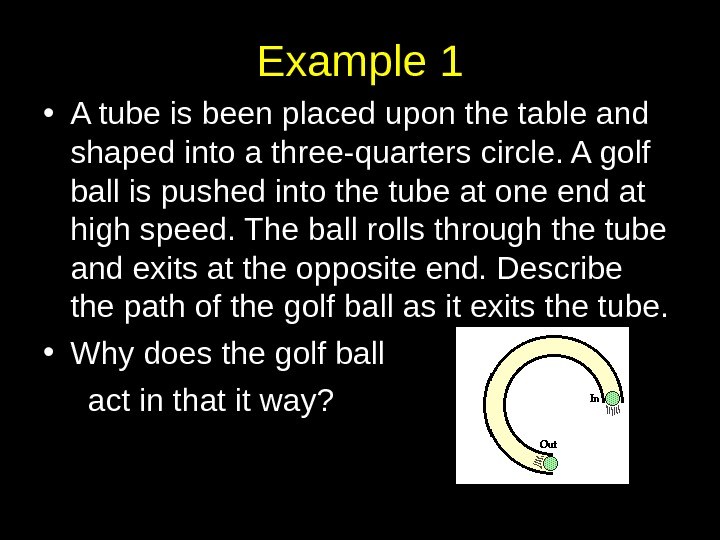Example 1 • A tube is been placed upon the table and shaped into a three-quarters circle. A golf ball is pushed into the tube at one end at high speed. The ball rolls through the tube and exits at the opposite end. Describe the path of the golf ball as it exits the tube. • Why does the golf ball act in that it way?An LP rotating at 33. 3 rpmExample 2 A 5 tenge coin is placed at the edge of a record, i. e. , an old fashioned LP, of diameter d = 30 cm. The record makes 33. 3 revolutions per minute. a) draw a free body diagram of the forces acting on the coin b) find the angular speed of the record c) if the coin is 2 g and the maximum frictional force provided by the LP is 6. 0 x 10 -4 N, calculate whether the coin will stay on or slip off.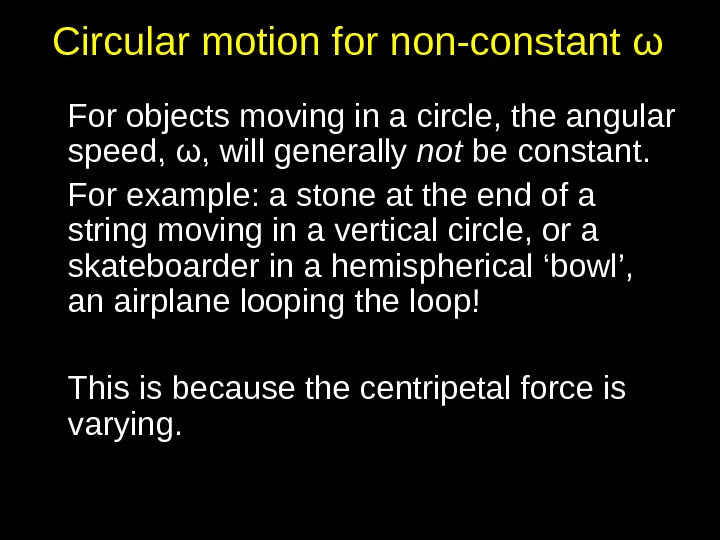Circular motion for non-constant ω For objects moving in a circle, the angular speed, ω, will generally not be constant. For example: a stone at the end of a string moving in a vertical circle, or a skateboarder in a hemispherical ‘bowl’, an airplane looping the loop! This is because the centripetal force is varying.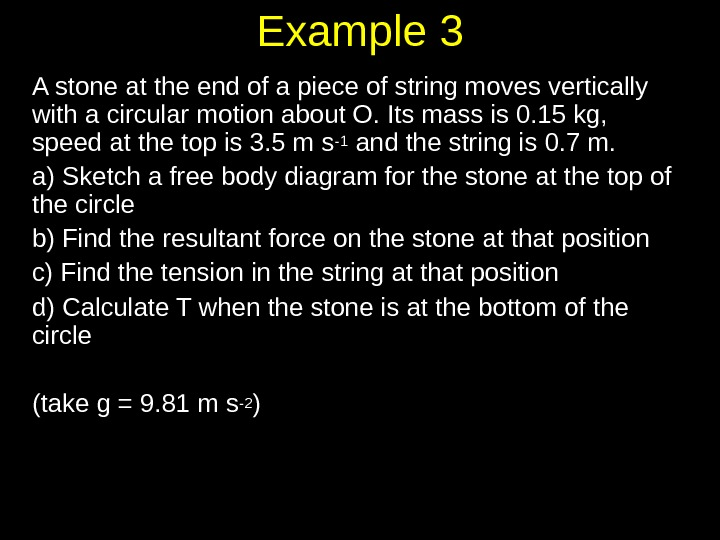Example 3 A stone at the end of a piece of string moves vertically with a circular motion about O. Its mass is 0. 15 kg, speed at the top is 3. 5 m s -1 and the string is 0. 7 m. a) Sketch a free body diagram for the stone at the top of the circle b) Find the resultant force on the stone at that position c) Find the tension in the string at that position d) Calculate T when the stone is at the bottom of the circle (take g = 9. 81 m s -2 )CHECK LIST READING Adams and Allday: 3. 28, 3. 29 At the end of this lecture you should • Be able to define angular speed, centripetal acceleration, centripetal force • Appreciate why an object moving in a circle must have a force acting on it • Understand the direction and nature of both the centripetal force and centrifugal force • Understand how circular motion can arise in a number of different situations in which the force applied to an object is perpendicular to the direction of the object’s motion • Appreciate the difference between circular motion of uniform angular speed and circular motion in which the angular speed may be varying • Be able to give examples of such situations and perform calculations to work out various properties of the circular motion in these different cases